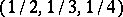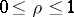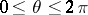##### Actions

A paradox connected with an inaccurate formulation of the initial assumptions in solving problems in probability. Noted by J. Bertrand . Bertrand's problem is concerned with the probability that the length of a chord, chosen at random in a disc of radius one, is larger than the side length of the inscribed equilateral triangle. Bertrand singled out three various values of the unknown probability, depending on the parameters characterizing the location of the chord. (In the first case by the distanceto the centre of the circle and the anglebetween the normal to the chord and the-axis; in the second by the angular coordinatesandof the points of intersection of the chord with the circumference of the disc; in the third case by the Cartesian coordinatesof the base of the normal dropped from the centre of the disc. In all three cases the coordinate origin coincides with the centre of the disc.) It was shown by H. Poincaré  that the origin of the paradox is due to the fact that in each of the three cases the respective pair of parameters is assumed to be uniformly distributed in the given zone, so that threeproblems are in fact solved. If the distribution of some pair (say,and) is fixed, the distribution of all other parameters can be uniquely calculated (and is not necessarily uniform, even ifandare uniformly distributed). From the geometrical point of view, the most natural assumption is thatandare independent and uniformly distributed in the interval,(cf. ).